alternative to curve-fit (Prony)

Member Posts: 35

I am modeling a visco-elastic behavior. I insert the Shear Data (Shear Modulus-Time) for temperature of 20° and 30°C and I get the Prony Shear Relaxation data after solving the curve fit. But as mentioned on a previous post, I think that curve fitting is not working properly; why do I think so?

• Because the displacement when the Environment Temperature (under Static Structural) is set to 20°C is higher than that of 27°C, meanwhile the shear modulus at input data is decreased when the temperature is increased.
• Prony parameters follow no trend

Is there any alternative way to calculate the visco-elastic behavior without using curve fit?

• PittsburghPosts: 187Ansys Employee
edited August 2018

There are two ways to represent temperature dependence in viscoelastic materials.

1. Multiple sets of Prony coefficients with each set representing relaxation at a different temperature (i.e. 20C ..30C).  In this case, you should curve fit the relaxation data for each temperature separately.

2. If the material exhibits TRS (thermo-rheologically simple) behavior, you can curve fit a master curve at one temperature and include a shift function to simulate relaxation at the other temperatures.

• edited August 2018

Hello jjdoyle,

thank you for the reply, but I would really appreciate if you could elaborate the 1st more.

What do you mean to curve fit separately? I input the relaxation data at 'Shear Data - Viscoelastic' for 20 and 30°C [Fig]. When I do curve fitting, Prony coefficients are generated for both temperatures automatically. How do you do them separately? And these coefficients will be the same even if I solve the curve fit for 20 and 30°C separately in separate Engineering Data standalone systems.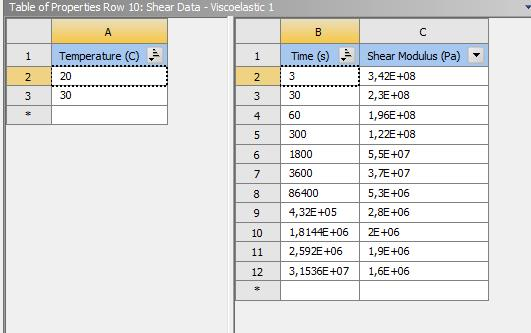• PittsburghPosts: 187Ansys Employee
edited August 2018

If you are not using a shift function and you read in multiple sets of relaxation data for different temperatures, you should get a different set of prony coefficients for each temperature.    If you include a shift function as part of the curve fitting, you need to specify what the master curve is.

I suggested just trying to curve fit one temperature because that is often easier to trouble shoot when the curve fitting is not working out.

The images below show a successful curve fit with 20C using the data you provided.  I used 7 terms and seeded the relaxation times to go from 1 sec to 1e7 because that sometimes helps produce a better fit.  It doesn't matter if the relaxation times for each term are not in ascending order.  Just think of it as seven terms in a mathematical prony expression to represent the relaxation of the modulus over time.  Also, I think there might be more then one solution to this problem.  In other words, using different numbers of terms and using default seed values might produce another combination of prony terms that is equally valid for matching the relaxation behavior.

I hope this helps.

************************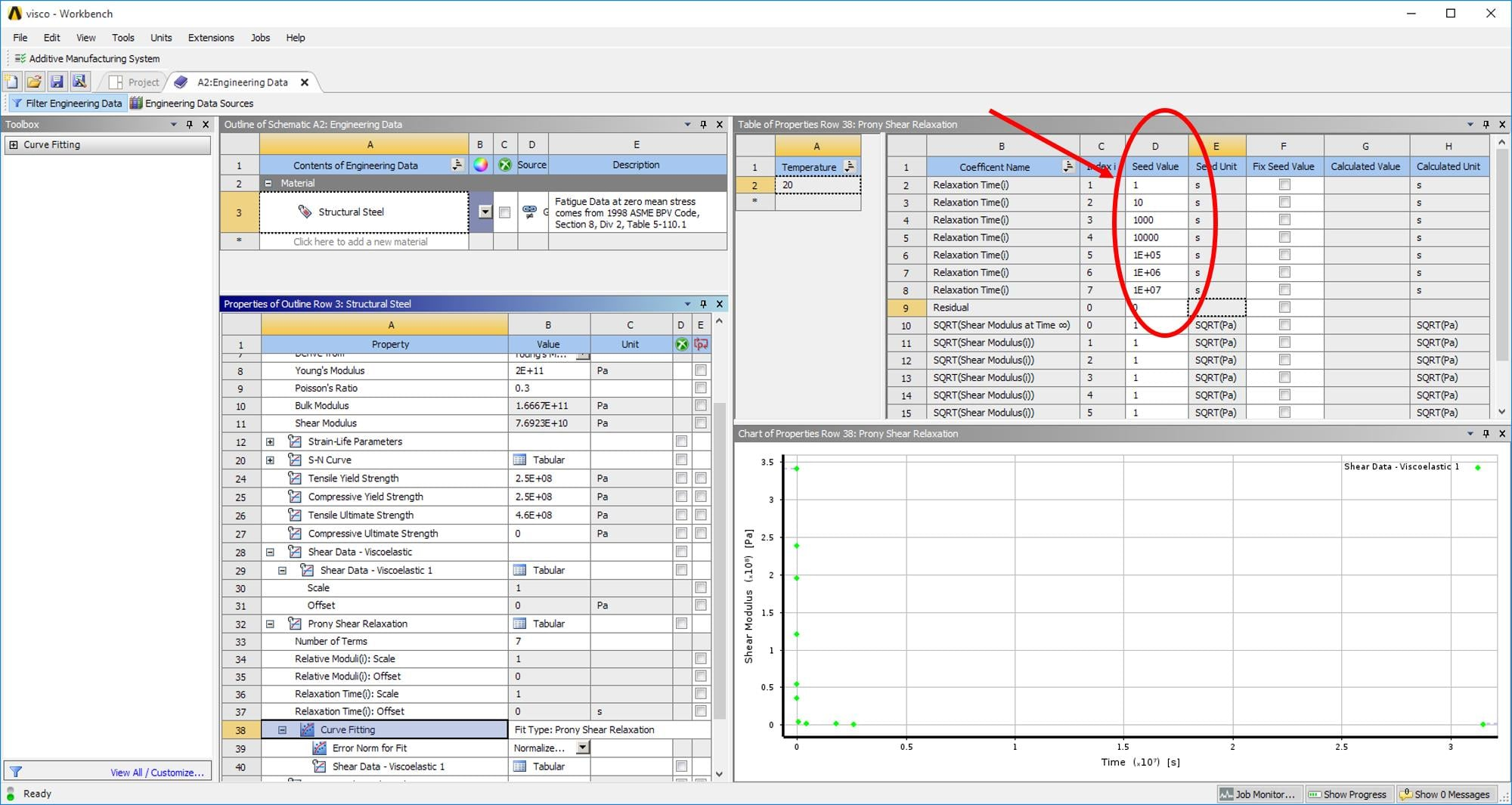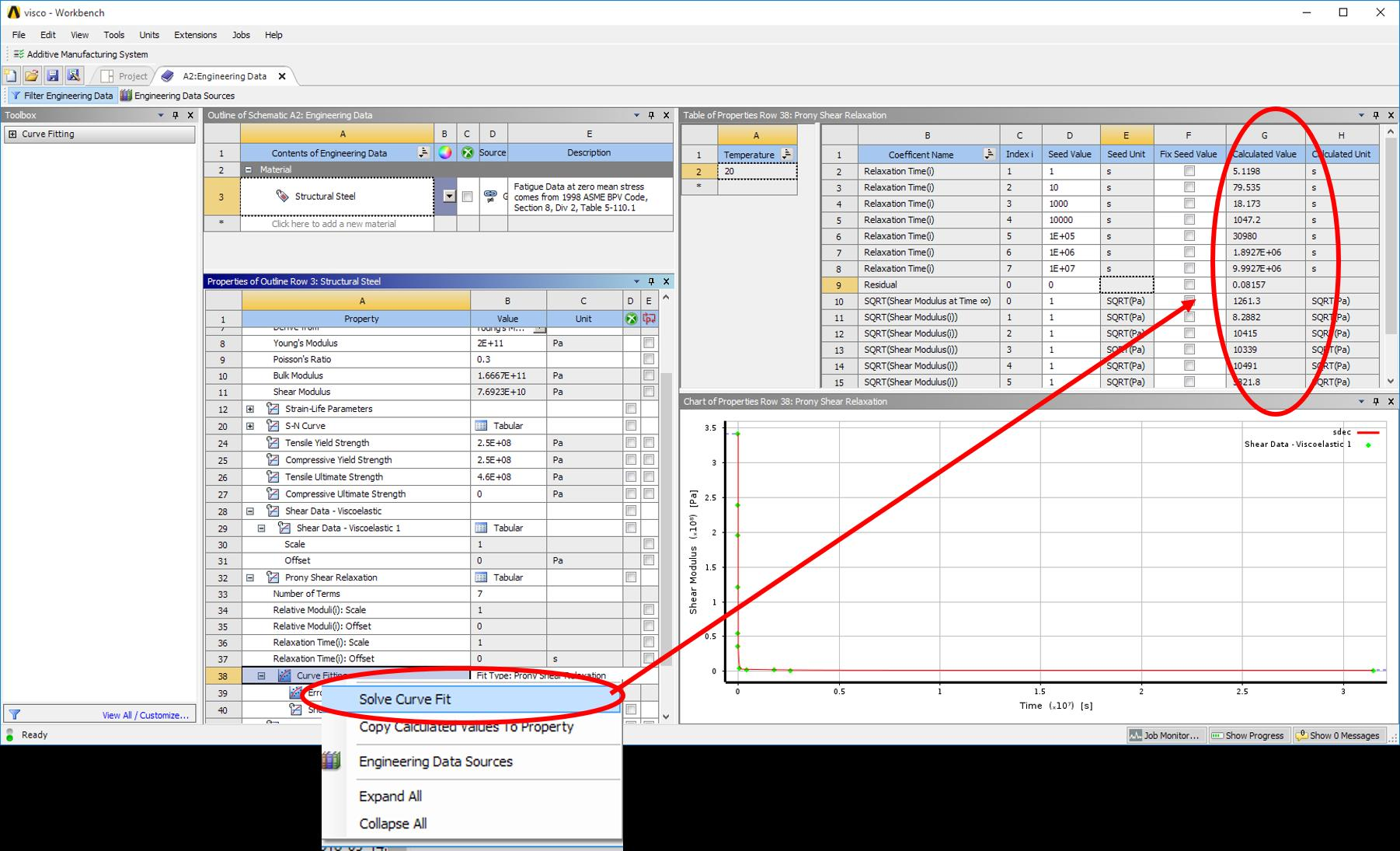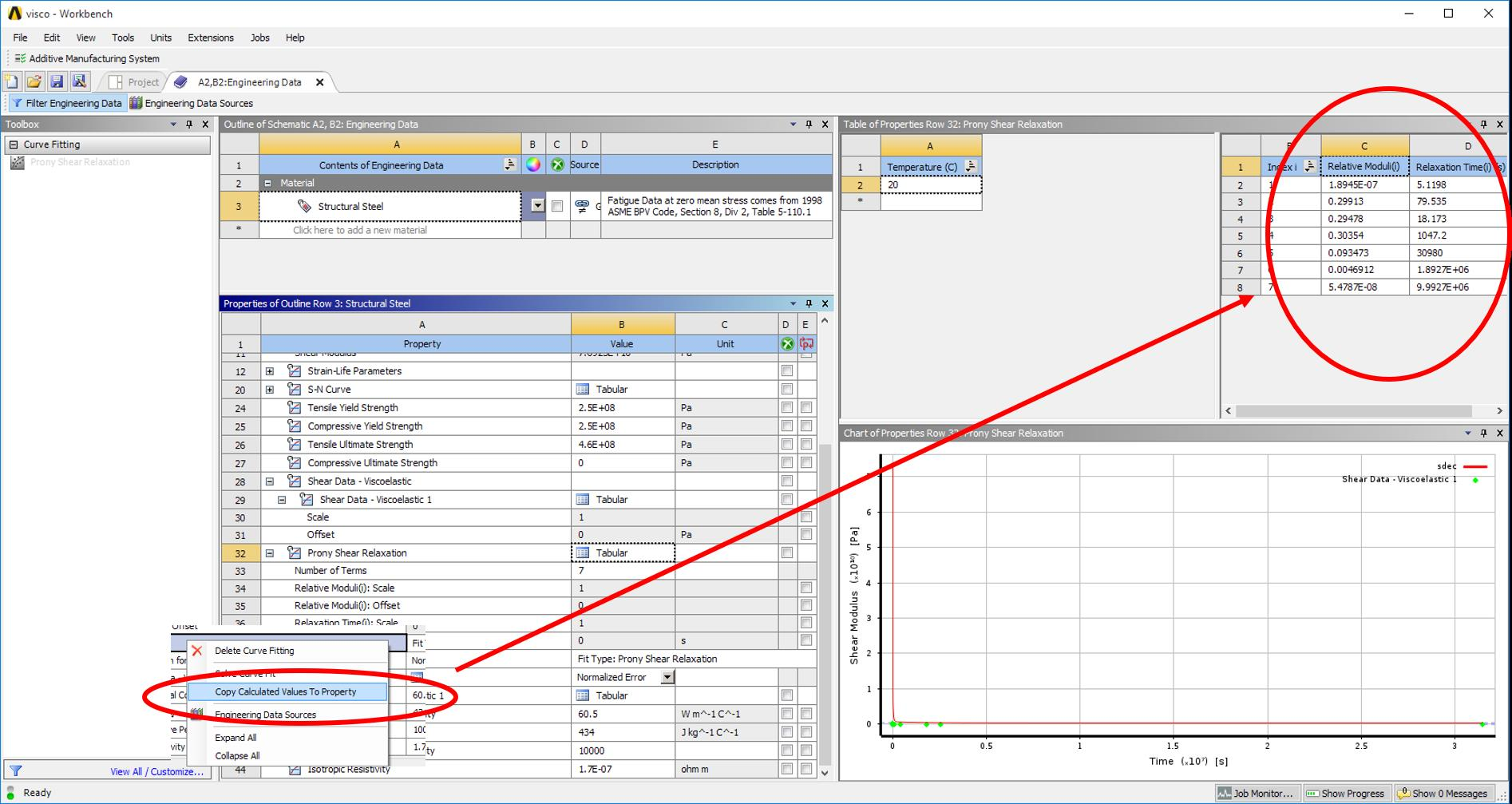• edited August 2018

jjdoyle,

thank you for the reply. I researched a bit more about how this works and now I understand that terms do not matter to be in order.

The tip for seeding relaxation time from 1s to 1e7 helps because the fit is better now; thank you. However, if we use a logarithmic scale for axes of the graph we can still see that the fit might need improvement.

And I need to understand some concepts to proceed with that:

• What does a Seed Value represent?
• Or what is the Seed value of Residual.
• Is SQRT(Shear Modulus at time infinite) the square root of G infinite?
• Do you have any other tips on what to change, like SQRT(Shear Modulus(i))?

I could not find any document to explain these in customer portal as well.

Regards,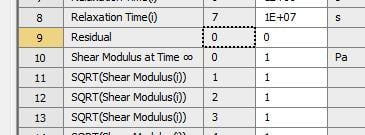• edited August 2018

Jonsys,

Curve-fitting is usually a nonlinear regression optimization problem which requires an "initial guess" (or the seed value) for the parameters that are being calculated. Highly nonlinear systems such as this one tend to have multiple local minima and the choice of initial guess can influence the local minima that the optimization algorithm will find. So, it is necessary to use physically realistic seed values for relaxation times. Just getting the order of magnitude in the ballpark can do the trick. One way of physically interpreting the Prony series is: the stress relaxation happens over a time and there may be multiple relaxation chains (mathematically, Prony terms) that may be contributing to this relaxation. So, a Prony term (Gi, Ti) may be interpreted as Gi fraction of the total stress relaxases over a time of Ti. This is a very loose interpretation of the math but it'll help in understanding the Prony series better.

For curve fitting, look at the timeline of your data. E.g., if you have data for a time of, say, 10^6 sec, and if you're using 3 Prony terms, then use seed values for time constants evenly distributed along that timeline (1s, 1e3s, 1e6s) or if you see a sharp dip at around 1e4 s then (1s, 1e4, 1e6) would be a good start. This way you'll have better chances of capturing the relaxation terms that are prominent along the timeline.

In general, you need not assign seed values for shear modulus and residual so don't tinker with them for now.

• edited August 2018

bsista,

that is a great explanation, thank you.

• Based on the relative moduli and relaxation time, I am calculating time dependent Shear Modulus just to compare input and output comming from the curve fit. From [Fig 1] it can be seen that the difference is still high, even though for the curve fit I used physically realistic seeded values as suggested.
• And alpha infinite (long term relative moduli) comes from G infinite/G0 [Fig 2]. What value is by default chosen by ANSYS because I do not input into the software a value for long-term modulus.

Regards,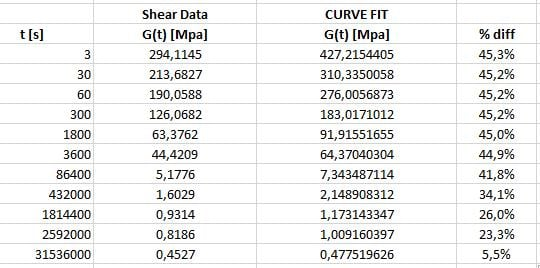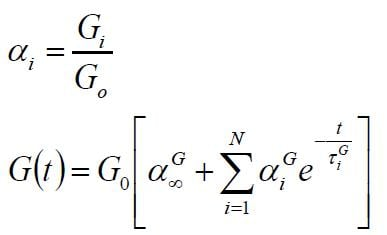• edited August 2018

Ah, don't do that!While performing the Prony series curve fitting, the material stiffness is not considered since we deal with normalized numbers. I don't know exactly how ANSYS does this but when I wrote my own script for curve fitting, I normalized the relaxation stress curve by dividing the stress values by the peak stress (stress at t~0s). ANSYS might be doing something similar, did you try that?

We generally need two sets of data for fully calibrating viscoelastic materials

• uniaxial tension/compression (and other modes of deformation) for calibrating the elastic properties
• stress relaxation data for calibrating Prony series properties.

Both the properties are calculated separately via curve fitting.

• edited August 2018

bsista,

What exactly to try? After normalizing the relaxation stress where should I use the values? By normalizing relaxation stress, do you mean to normalize shear modulus?

Note that I am using the relative moduli and relaxation time that is solved by ANSYS curve fit. I substitute these values into the formulas mentioned in my previous comment (also stated in ANSYS documentation) and get Shear modulus dependant on time G(t). So I am not doing any curve fit or Prony coef calculation on my own.

Regarding the data, I have instantaneous shear modulus, poisson's ratio and time dependent shear modulus - I guess this is enough right?

Regards,

• edited August 2018

Where are you getting your instantaneous shear modulus from? What I was suggesting is that the G0 (instantaneous shear modulus) in the equations that you shared should be the peak shear modulus from the time-dependent shear modulus curve. Even if you're getting the G0 value from some other experiment, use the max. value from the curve for verifying the fit since the G0 value that you may have from other experiment is not used during the curve-fitting stage.

Regarding the data, what you have is sufficient to define the viscoelastic material. The instantaneous shear modulus and the Poisson's ratio must be input under Isotropic elastic properties and the normalized relaxation constants and their time constants under viscoelastic behaviour.

• edited August 2018

I mostly get G0 from experimental results. Yes, G0 is always the highest value.

I did some further tries and now the curve fit works perfectly.

Thank you bsista and jjdoyle for suggestions.

• edited October 2018

Hello everyone,
Hello jonsys,

I'm a student. I need some help!

I have a thesis about Creep of concrete. I researched about it and i found Creep ralated to Viscoelastic. Can you explain me how to implement it?

I have read your comment, and I see my problem as in this discussion, using data you provided:
We had Shear Modulus from the experiment [Fig.1]. So why do we have to find G (t) in Relative Moduli and Relaxation Time [Fig.2]?
How does Number of Terms affect analysis (Ex: What is different between 3 terms and 4 terms)? Why do we have to choose 1s, 1e3s and 1e7s without the other number [Fig.3]?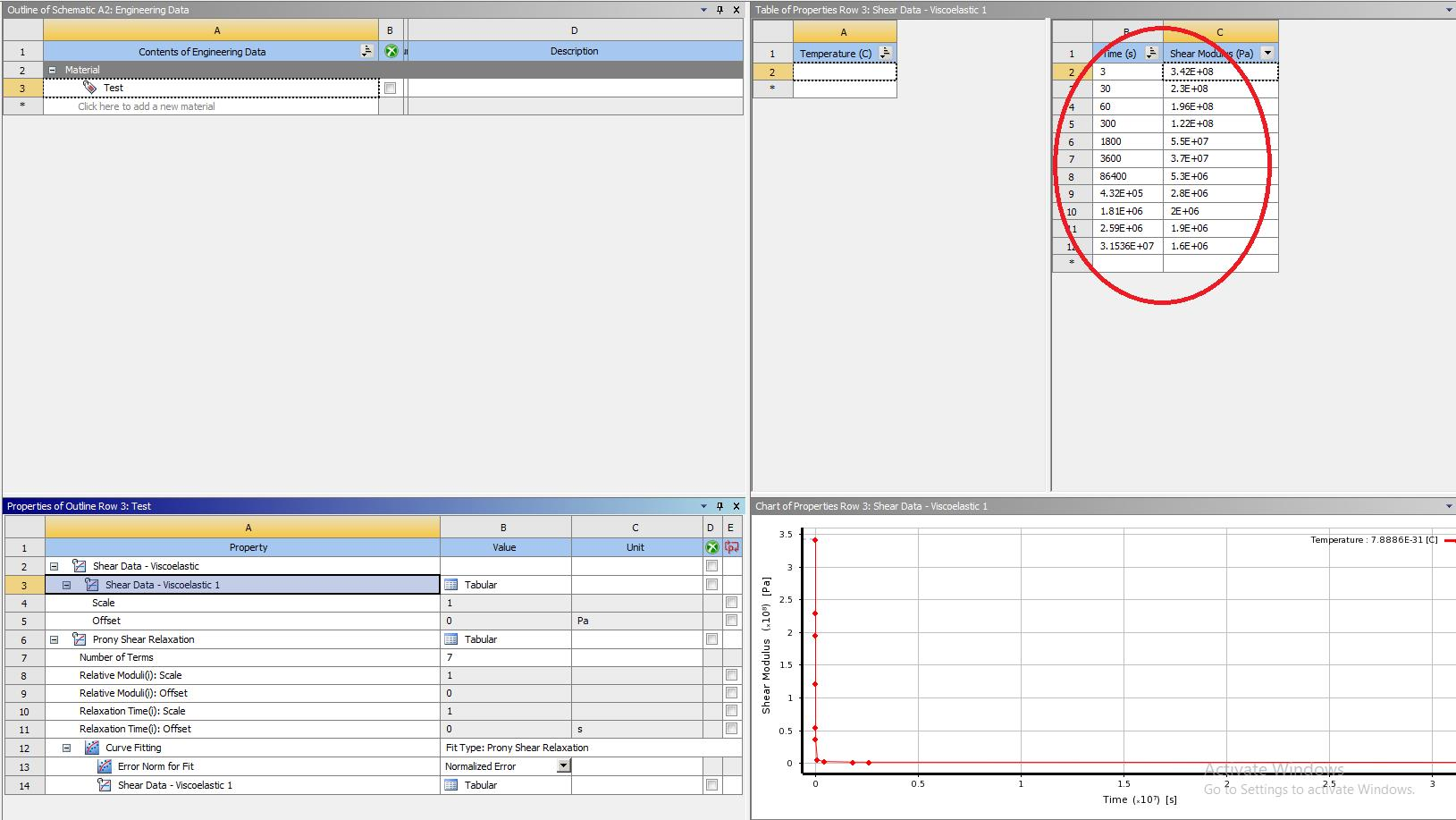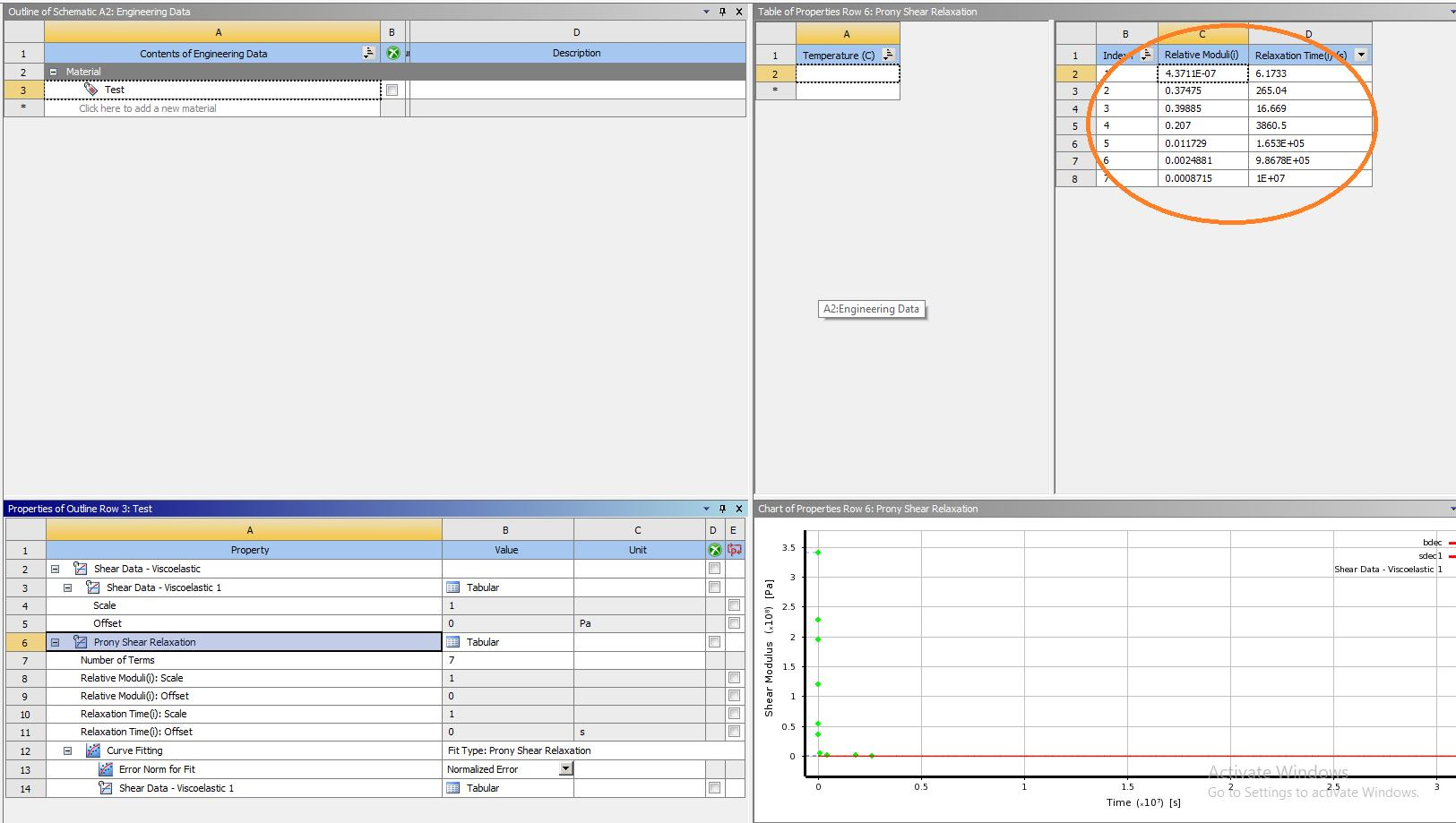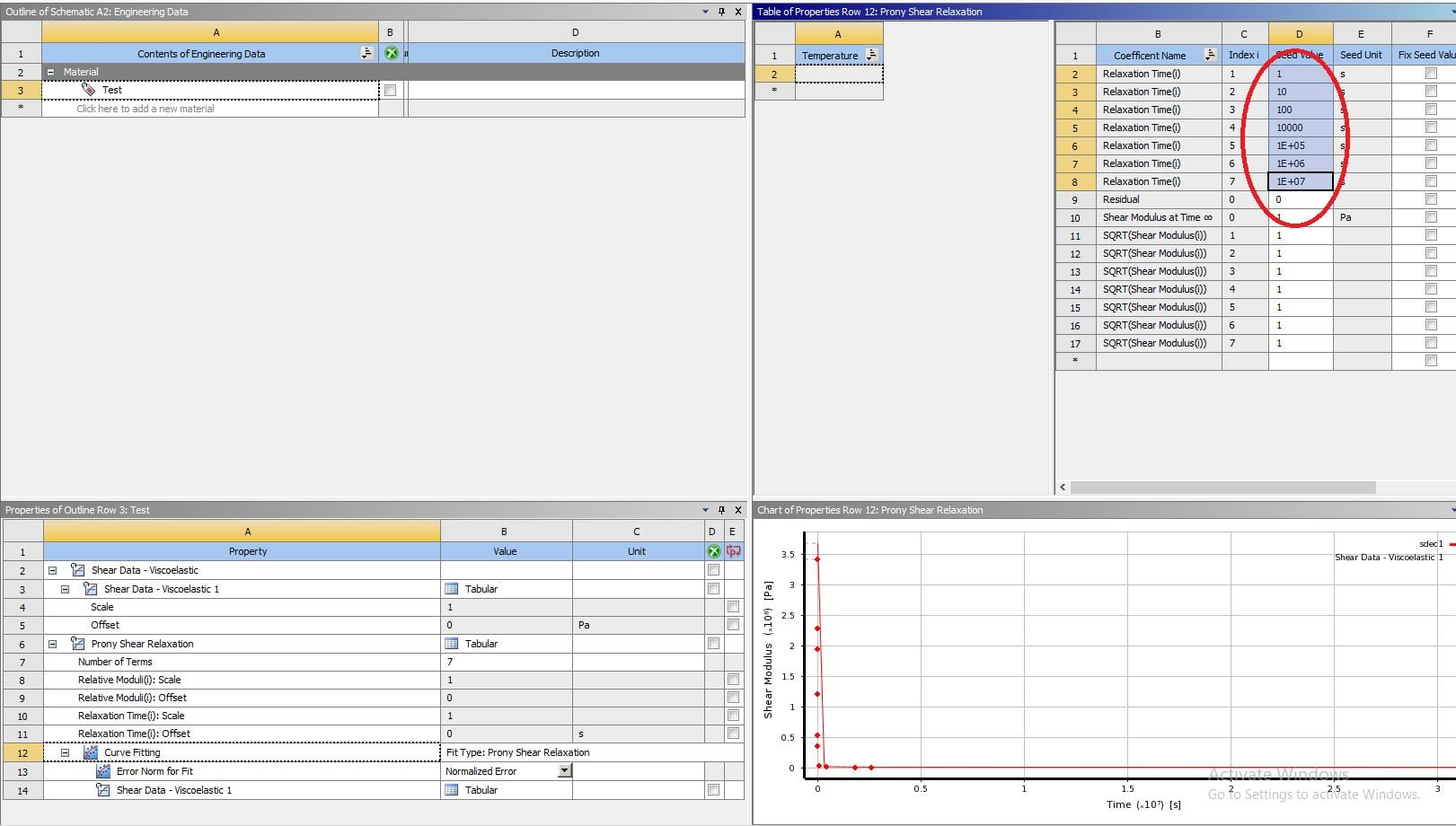Thanks in regards.

• edited October 2018

Hello Sangrey,

It is necessary in ANSYS to use Prony shear coefficients (That's how Ansys works). I guess the reason is that with those coefficients, we have a function (a curve) for Shear Modulus - Time relation, meanwhile from experiments we only have some discrete data.

Regarding 'what is different about 3 and 4 terms' check bsista comment on this post.

I am not sure if you have to use creep options, but if yes then check this from Peter.

Firstly, Fig 3 represents also the values in between and therefore 7 Prony parameters. bsista said that we can use 1s, 1e3s and 1e7s to have only 3 prony shear parameters and still have a good distribution of time (and therefore a better curve fit).

I hope it helps a bit.

• edited October 2018

jonsys,

(i) I don't use Isotropic Elasticity to define Young's Modulus(E), Poisson's Ratio(v), Bulk Modulus and Shear Modulus, specifically G, but still find G-inf [Fig.1]. Have you tried yet?

(ii) The line in Curve-fit [Fig.2] is different from the line in Prony Shear Relaxation (almost straight line) [Fig.3]. Is that a problem?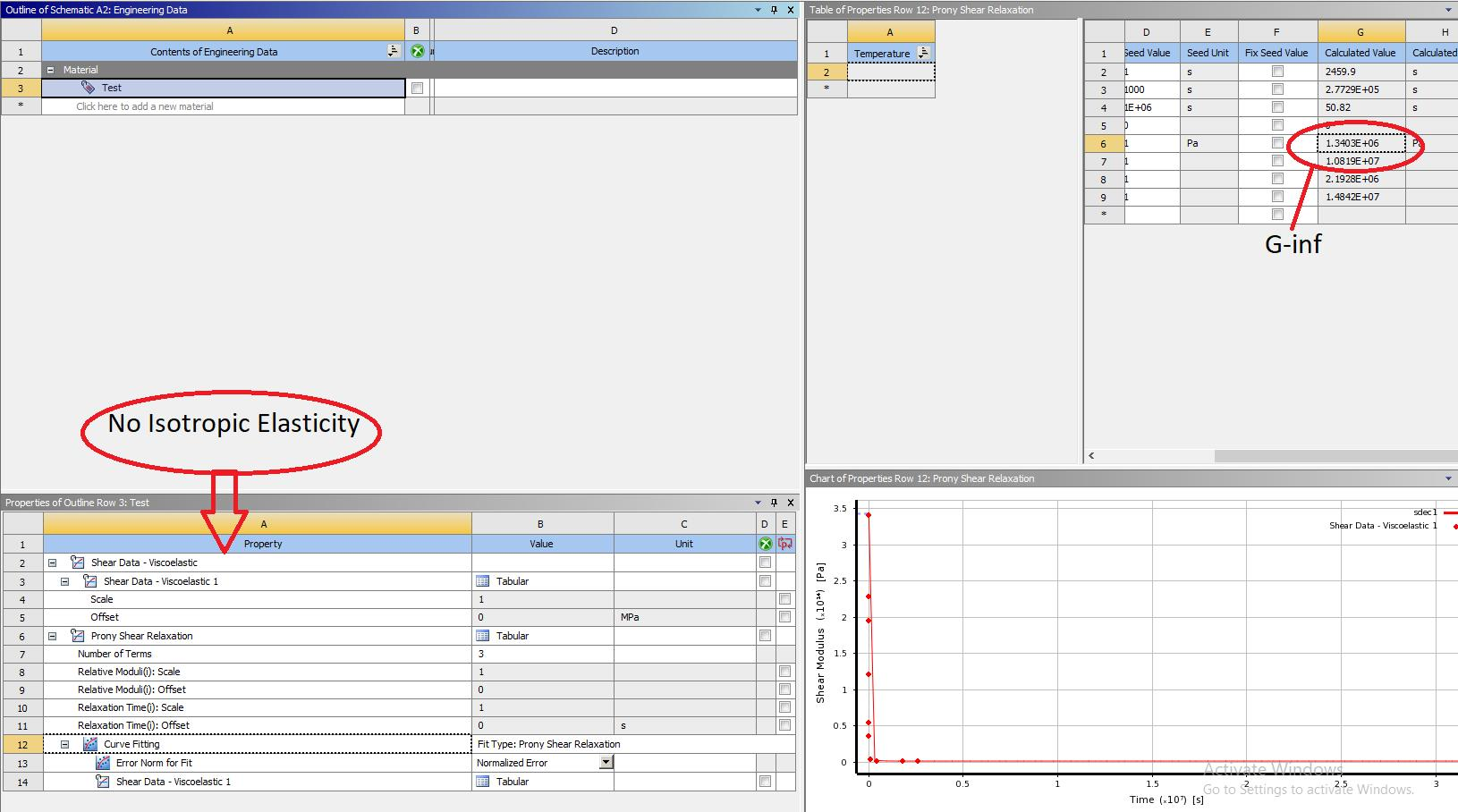Fig.1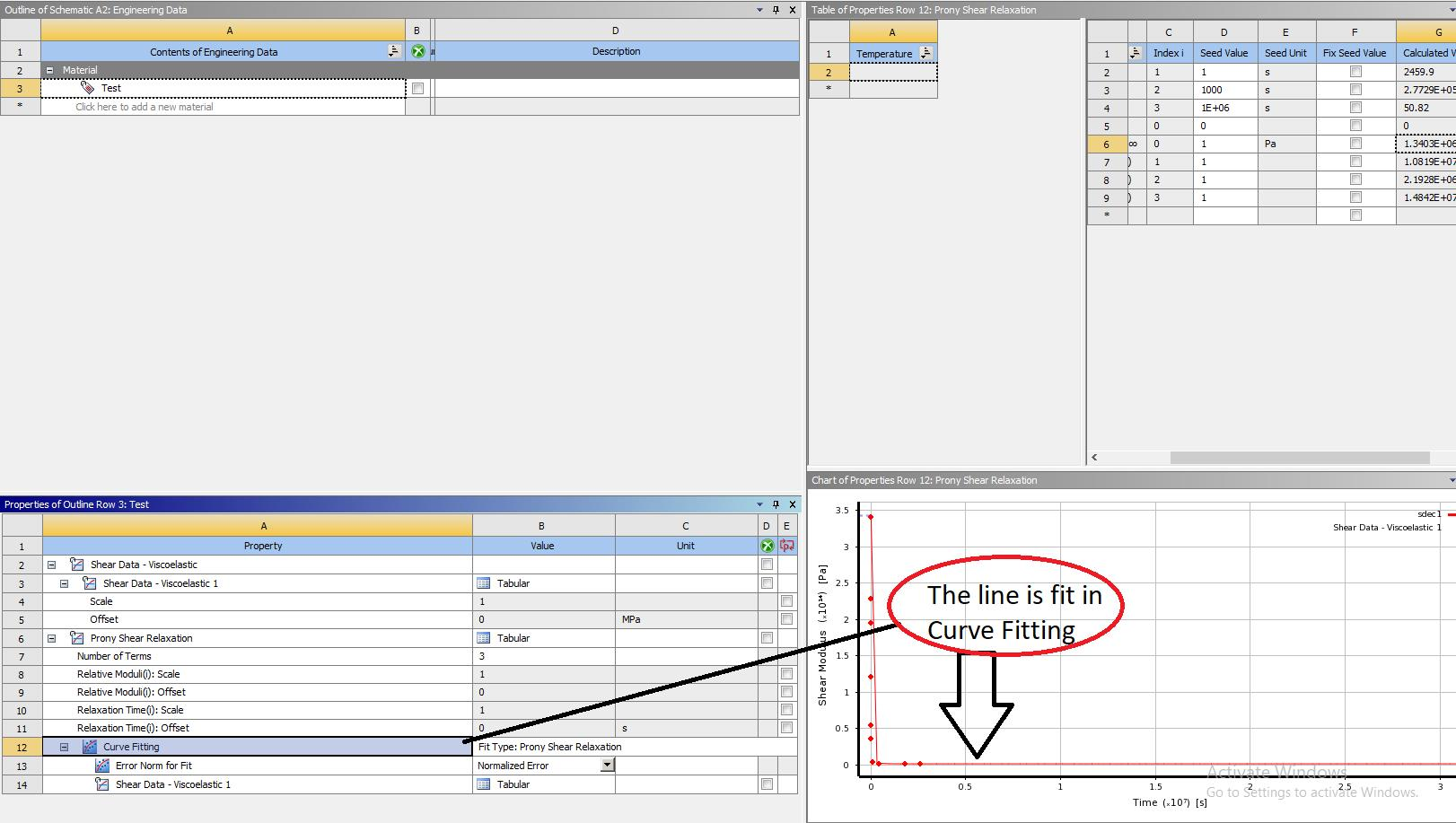Fig.2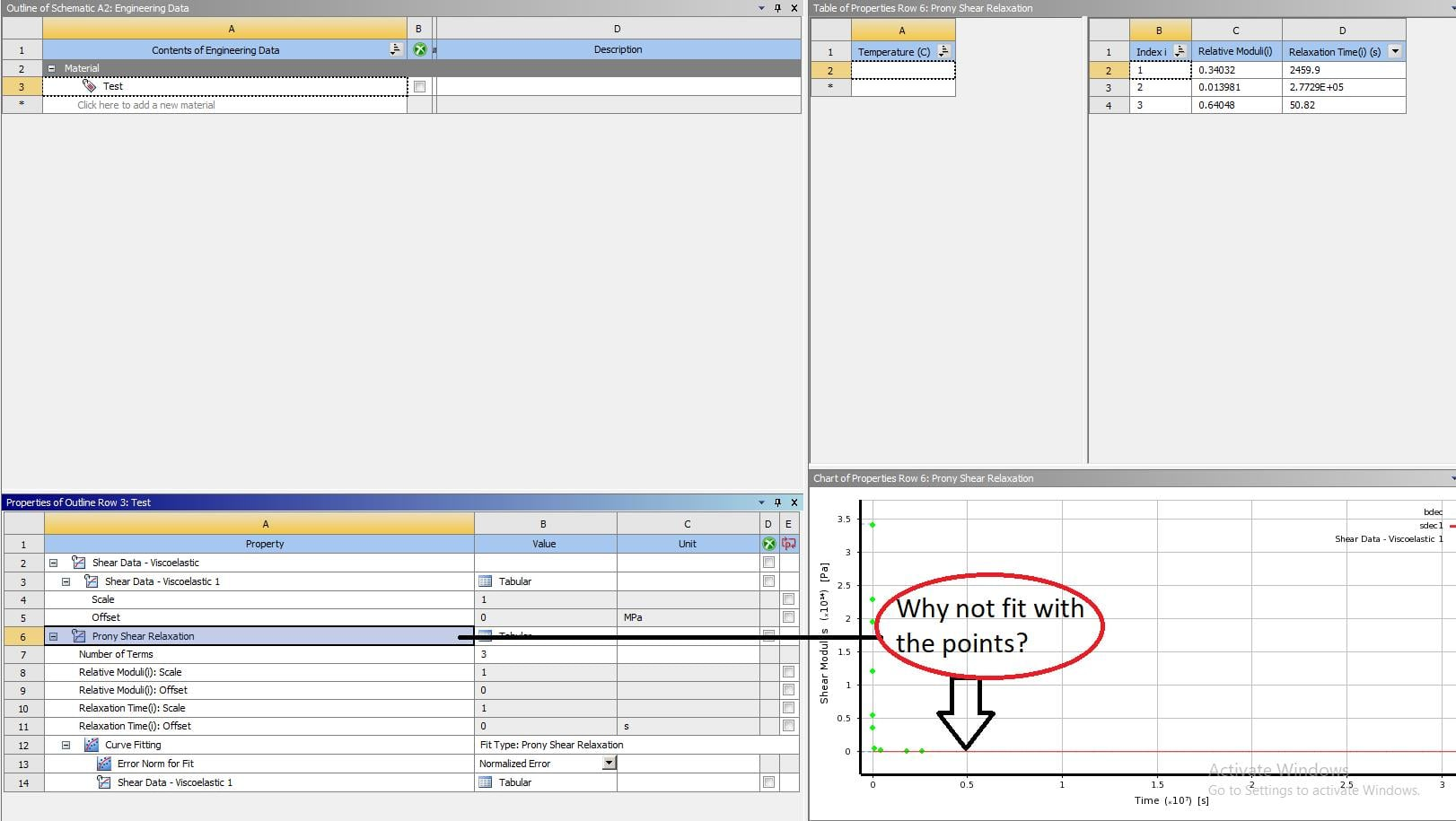Fig.3

• edited October 2018

Hello Sangrey,

I am not sure I understood correst your first questions; but I haven't tried to define G inf (if that's what you asked)

2. There is a problem in visualization if you are using other version of ANSYS rather than v19.0 (.1 or .2)
If the time is big in your case, right click on the x-axis of the graph and make it logarithmic scale - it is easier to judge the curve fitting.
One way to check curve fit is to build yourself in excel (or whatever) the curve using prony coefficient generated from ansys and compare them with G(t) of experimental results. The formulas are in ANSYS documentation at Visco-elastic section (I also put them in my previous comments)
Important note: G0 has a considerable effect in the results.

Regards

• edited October 2018

Hello jonsys,

Firstly, I really appreciate your help with my project! And I sorry that my first question was unclear.

Would you mind if I request your email or social network account? to make friends. Perhaps we can help each other in another project.